Next: BURG SPECTRAL ESTIMATION Up: Waveform applications of least Previous: Waveform applications of least

# PREDICTION AND SHAPING FILTERS

A data wavelet is given by b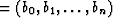.We plan to construct a filter f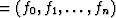.Filtering is defined in this way: When data b go into a filter f, an output wavelet c is produced according to the following matrix multiplication.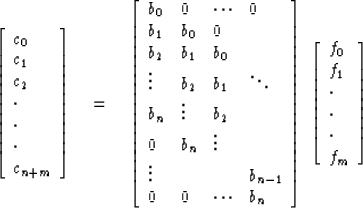(1)
This operation is often called complete transient convolution. It is the same as identifying coefficients in a polynomial multiplication.

Now we introduce another wavelet d which will have the same number of components as c. We call d the desired output of the filter. We saw that c is the actual output. The actual output c was seen to be a function of the input b and the filter f. The problem now is to determine f so that c and d are very much alike. Specifically we will choose f so that the difference vector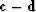has minimum length squared (in n + m + 1 dimensional space). In other words, we use the method of least squares to solve the overdetermined equations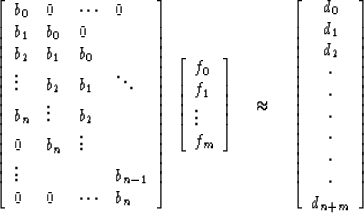(2)
Using the quick-and-dirty" method of the previous chapter we merely premultiply (2) by the transposed matrix. The result is a Toeplitz matrix of the form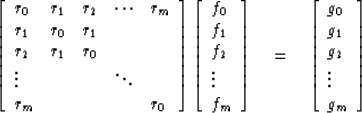(3)
where rk is the autocorrelation of the input xk and gk is a crosscorrelation of the input xk with the desired output dk. For computation techniques see Chapter 7.5.

The formulas of this section may also be used to attempt to predict a time series from its past. For example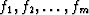is a prediction filter of xt+10 from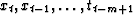if we solve by least squares the equations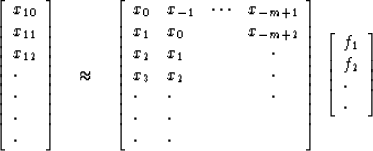(4)
The matrix in (4) may be continued downward for as far as one has data. In an application, the range of t in (4) would be over past values of t. Then, after solving the equations for the filter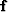it would be hoped that the character of the time series was such thatcould be used to predict future values of the time series which had not gone into the equation defining.

If the matrix of (4) is very much higher than it is wide, it may be desirable to treat the end effects differently. If one uses instead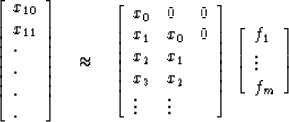(5)
one finds that the least-squares normal equation has a Toeplitz matrix whereas for (4) the matrix in not Toeplitz. As the reader is aware, the Toeplitz matrix has many advantages, both theoretical and computational.

Of special interest is the filter which is designed from the equations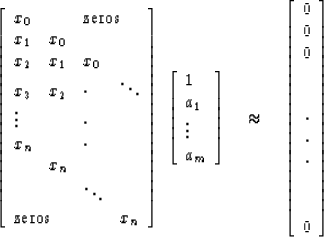(6)

Such a filter is called the prediction error filter for unit span because the ak operate on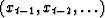attempting to cancel xt. Thus, the ak on thegives the negative of a best prediction of xt, based on.The normal equations implied by (6) are the square set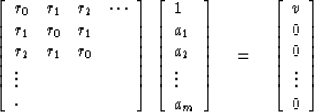(7)
It may be noted that the calculation of a prediction error filter depends only on the autocorrelation of the time series and not on the time series itself. As we have seen (from ()), the solutions to these equations are coefficients of a minimum-phase polynomial.

Solutions to Toeplitz equations when the right-hand side takes the more arbitrary form (3) are not generally minimum-phase, but the Levinson recursion may be generalized to make the calculation speedy. This is done in Section 7.5 on the multichannel Levinson recursion.

## EXERCISES:

1. Find a three-term zero delay inverse to the wavelet (1,2). Compare the error to the error of (2,1). Compare the waveform. An extensive discussion of the error in least-squares inverse filters is given in Reference 26. One conclusion is that the sum of the squared errors goes to zero as the filter length becomes infinite in two situations:

(a) Zero delay inverse if and only if the wavelet being inverted is minimum-phase.

(b) If the wavelet being inverted in not minimum-phase, the error goes to zero only if the output is delayed, that it,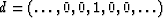.Calculate a three-term delayed inverse to (1,2), that is, try d = (0, 1, 0, 0) or d = (0, 0, 1, 0).

2. A pressure sensor in a deep well records upgoing seismic waves and, at some time t0 later, identical downgoing waves of opposite sign. Determine delayed and non-delayed least-squares filters of length m to eliminate the double pulse. (You should be able to guess the solution to large matrices of this type. Try filters of the form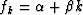where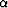and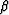are scalars.) What is the error as a function of the filter length?
3. Let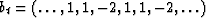.Find by least squares the best one-term filter which predicts bt, using only bt-1. Find the best two-term filter using bt-1 and bt-2. Likewise find the best three-term filter. What is the error as a function of time in each case?Next: BURG SPECTRAL ESTIMATION Up: Waveform applications of least Previous: Waveform applications of least
Stanford Exploration Project
10/30/1997